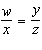Name:    Geometry Chapter 3 Practice Test - Similarity

1.

If, then ______.
 a.b. x = 7 c. x = 4 d. x = 3

2.

The geometric mean of 5 and 15 is ______.
 a.b. 75 c.d. 10

3.

If, then ______.
 a.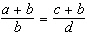c.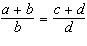b. ac = bd d.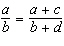4.

Given that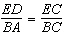, find AB to the nearest tenth. The figure is not drawn to scale.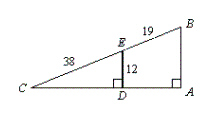a. 20.2 b. 14.7 c. 16.9 d. 18

5.

If two polygons are SIMILAR, then the corresponding angles must be _____.
 a. complementary c. congruent b. supplementary d. linear pairs

6.

If two polygons are SIMILAR, then the corresponding sides must be _____.
 a. proportional c. parallel b. congruent d. similar

7.

Given that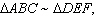solve for x and y.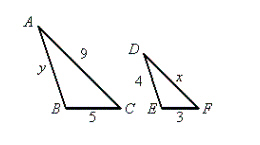a.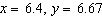c.b.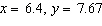d.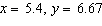8.

Which triangle is NOT similar to any of the others?
 a.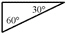b.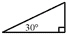c.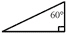d.9.

Triangles LMN and NWR are right triangles.What is the length of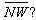a. 2.5 cm b. 10 cm c. 15.6 cm d. 14.4 cm

10.

One way to show that two triangles are similar is to show that ______.
 a. two angles of one are congruent to two angles of the other b. two sides of one are proportional to two sides of the other c. a side of one is congruent to a side of the other d. an angle of one is congruent to an angle of the other

11.

Shown below is an illustration of the ______.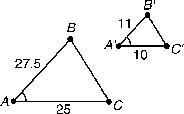a. AA Similarity Postulate c. SSS Similarity Theorem b. SAS Congruence Theorem d. SAS Similarity Theorem

12.

The postulate or theorem that can be used to prove that the two triangles are similar is _____.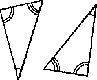a. SAS Similarity Theorem c. SSS Similarity Theorem b. ASA Congruence Theorem d. AA Similarity Postulate

13.

Given: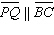. Find the length of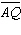.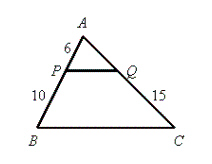a. 11 b. 9 c. 13 d. 6

14.

If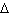ABC ~PBQ, then which of the following proportions is NOT true?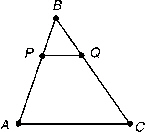a.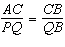b.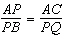c.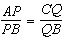d.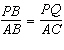15.

For the figure shown, which statement is not  true?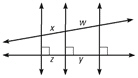a.b.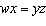c.d.